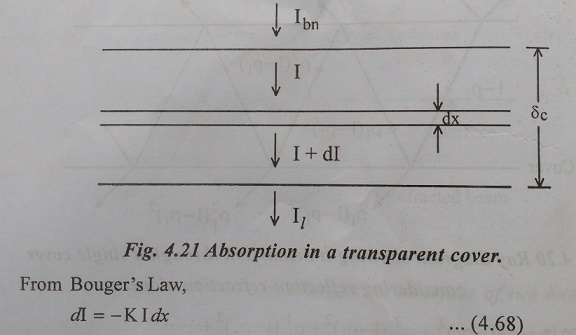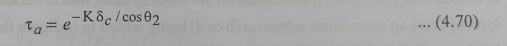Home | | Electric Energy Generation, Utilization and Conservation | Transmissivity of the Cover System on Flat Plate Collectors

# Transmissivity of the Cover System on Flat Plate Collectors

The transmissivity of the cover system of a collector can be obtained with adequate accuracy by considering reflection ŌĆō refraction and absorption separately and is given by the product form,

Transmissivity of the Cover System

The transmissivity of the cover system of a collector can be obtained with adequate accuracy by considering reflection ŌĆō refraction and absorption separately and is given by the product form,## Transmissivity based on reflection ŌĆō refraction

When a beam of light of intensity Ibn travelling through a transparent medium 1 strikes the interface separating it from another transparent medium 2. It is reflected and refracted as shown in Fig.4.19. The reflected beam has a reduced indensity Ir and has a direction such that the angle of reflection is equal to the angle of incidence. On the other hand, the directions of the incident and reracted beams are related to each other by SnellŌĆÖs Law which states that,## Transmissivity based on Absorption

The Transmissivity based on absorption can be obtained by assuming that the attenuation due to absorption is proportional to the local intensity (BougerŌĆÖs law) . Consider a beam of intensity ŌĆśIbnŌĆÖ incident normally on a transparent cover of thickness ŌĆś╬┤cŌĆÖ and emerging with an intensity ŌĆśIlŌĆÖ as shown in Fig.4.21.Where, K is a constant of proportionality and is called the extinction coefficient. It will be assumed to have a value independent of wavelength. Integrating over the length traversed by the beam, we haveIn case the beam is incident at an angle q1, the path traversed through the cover would be (╬┤c/cosq2), where qis the angle of refraction. Then Equ. (4.69) gets modified to the form,The extinction coefficient K is a property of the cover material. Its value varies from about 4 to 25cm-1 for different qualities of glass. A low value is obviously desirable.

Equ.(4.69) and Equ.(4.70) are derived for one cover. If there are ŌĆśMŌĆÖ covers the exponent in these equations would be multiplied by ŌĆśMŌĆÖ.